InvestmentTools.com | home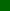If Stupidity got us into this mess, then why can't it get us out?Will Rogers (1879-1935)DJIA  Dow Jones Industrial AverageDJIA long term "log" chartDJIA from 1961Dow / Gold RatioDJIA  FuturesDJIA Index (recent) DJIA Point & Figure ChartDow / Silver RatioNotes & Links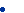More Equities Dow / Oil RatioDow / Real Estate RatioDOW TheoryDJIA CPI adjustedFlaws in the DJIA DJIA / RE RatioDow Jones Industrial Average (long term log chart).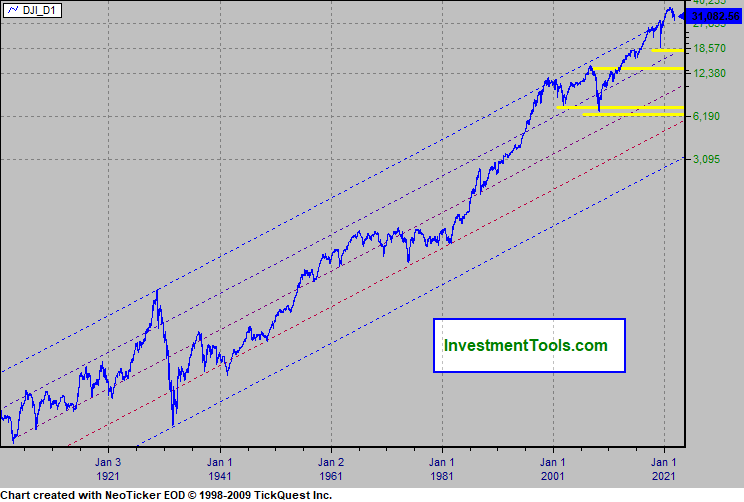DJIA (arithmetic chart), 200 day exponential average (red), 200 day rate of change (green).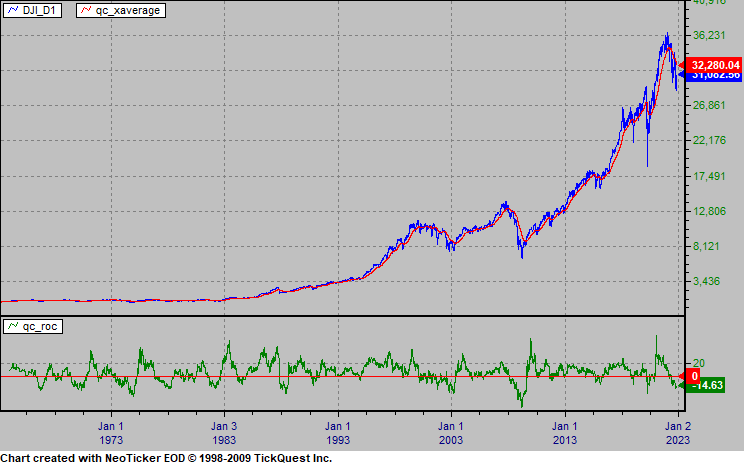DJIA Futures The Blue line is a Donchian channel, red line is a 5 day exponential average, green line is a 20 day exponential average. Vertical green bars MACD Moving average convergence divergence 5 and 20.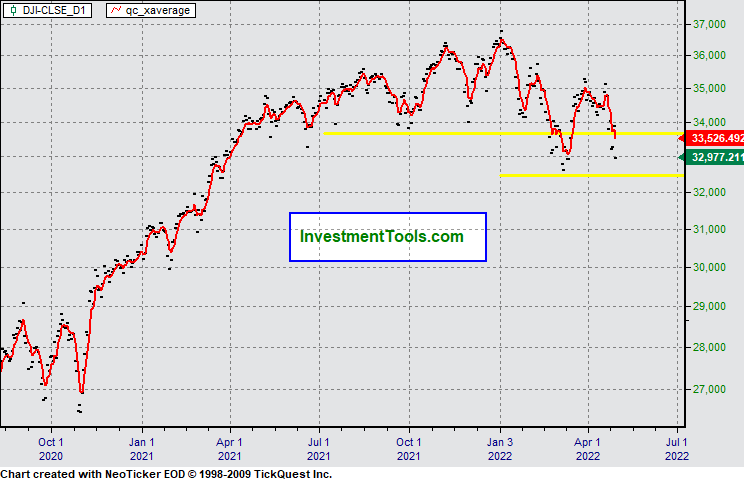Dow Jones Industrial Average adjusted by the CPI (Consumer Price Index)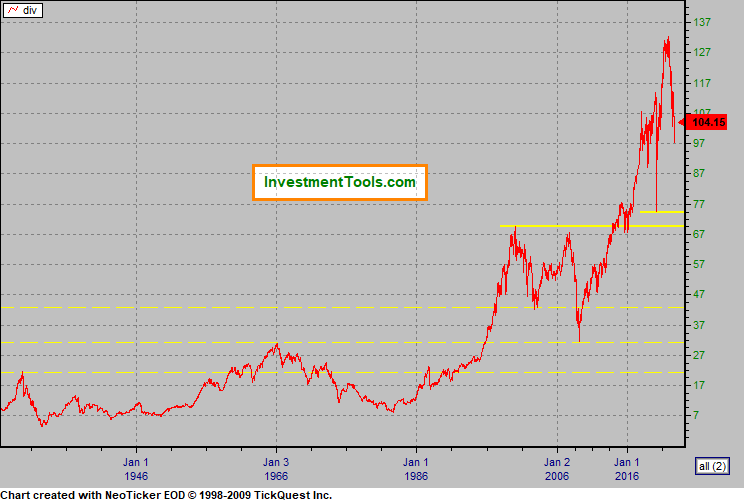DJIA Recent. The blue line is a 20 day Donchian channel,  green  line is a 20 day exponential average, yellow line is a 200 day exponential average.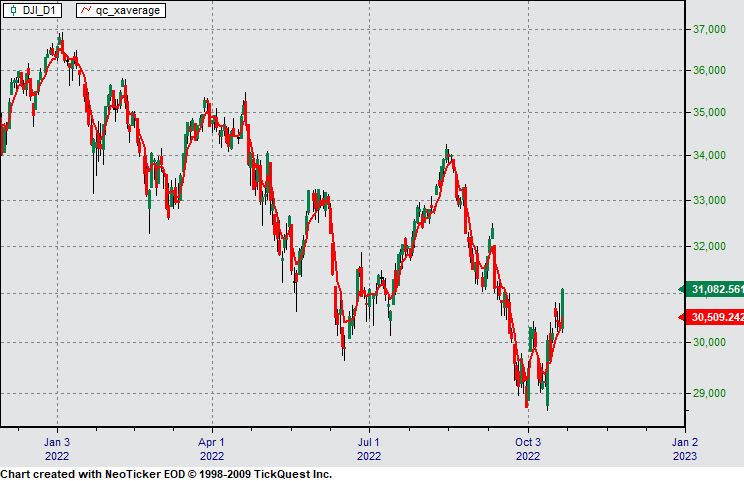DJIA Point & Figure Chart (Index) Box = 30 Reversal = 3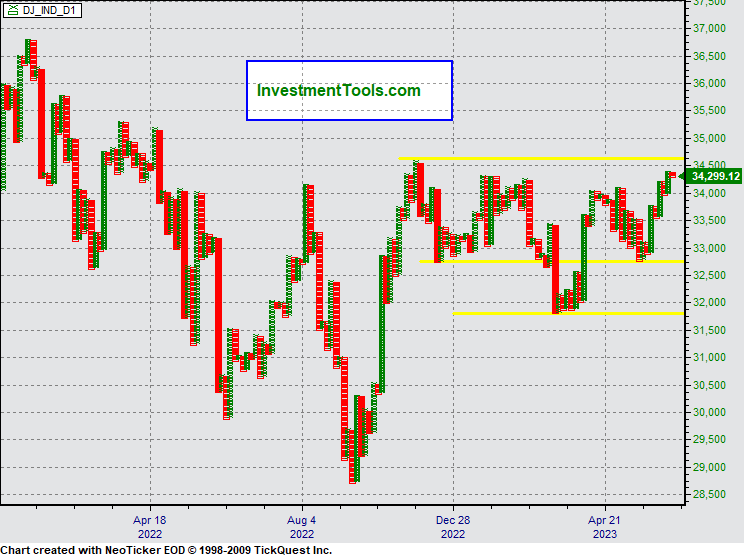Dow / Gold Ratio (DJIA divided by Gold \$ per oz price) 400 day xaverage yellow.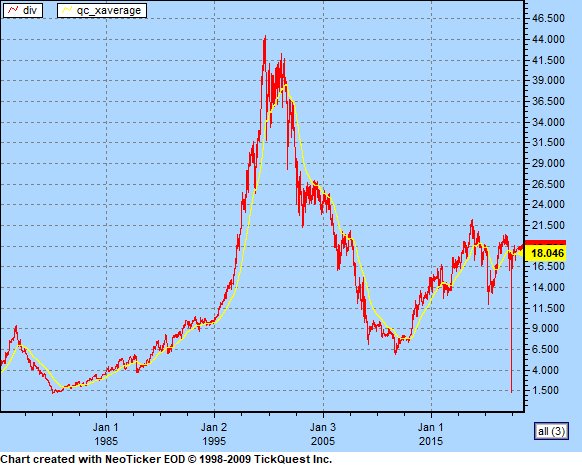How many shares of the Dow Jones Industrial Average does it take to buy the Average U.S. House? Average U.S. real estate price divided by Dow IndustrialDow / Crude Oil Ratio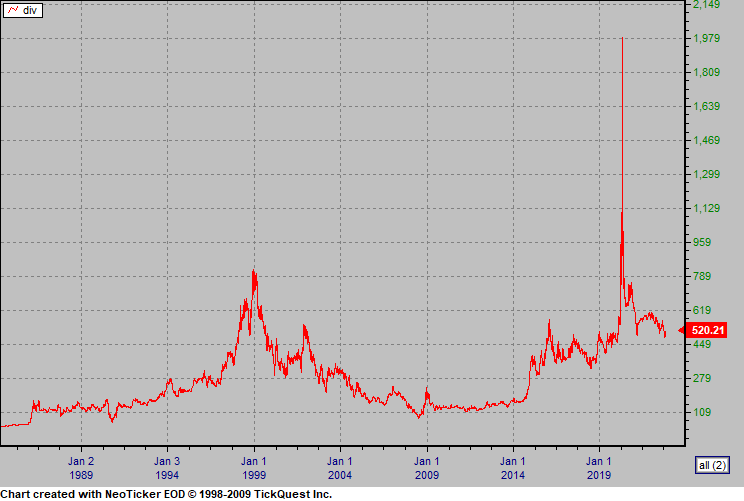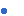Flaws in the major stock indices: The average dividend yield over the whole period was 4.87 percent. Ignoring this return leads to enormous understatements of the long run payoff to owning stocks. It would not be difficult to publicize a total return index rather than a stock price index. On a daily basis, the difference would be barely noticeable. However, over time horizons longer than three months, the difference becomes noticeable. Over decades, the difference becomes enormous. A value-weighted index of the Dow-components including dividend payments (VW-DOWD) would have closed at 233,060 points at the end of December 1998 had it started off in October 1928 at 239.43 points.